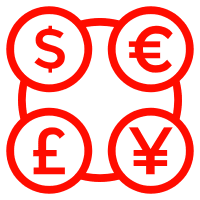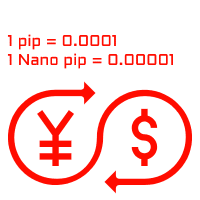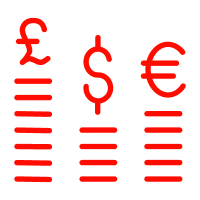# Forex pip explained – What does it stand for?

Pip in Forex trading stands for the percentage in point. It is a measurement of the minimum price movement in the Forex trading market. Depending on the currency pair that you are trading, the Forex pip might be different. For example, for the majority of Forex currency pairs, pip is the fourth number after the decimal point – 0.0001, while for currency pairs involving JPY, it is the second number after the decimal point – 0.01.

The Forex pip is the smallest possible amount by which the price of a currency pair can change. The main idea behind the size of pip is to protect traders from losing huge amounts of money from small changes in the market. Imagine if the pip was 10 basis points instead of what it is, in this case, even the tiniest change in the market would cause greater volatility in the value of the currency.

But, this is not all. There is much more that you should know about pip in Forex trading. So, follow our comprehensive guide to Forex pip size and learn everything that there is to know.

## Forex pips definition – Why is it important?As we have already said, pip is the incremental price movement, or change, of a currency pair in Forex. If you have been wondering what does pip stand for in Forex, you should know that sometimes pip is defined as the percentage in point, while others define it as the price interest point. Understanding how pip works in Forex is vital for every trader.

The number of pips that you generate or lose while trading Forex, can be either very profitable for you, or destructive for your position. The price change in Forex trading is, in most cases, very incremental, which is why a pip is such a little amount. To make everything easier for you to understand, we will continue by discussing the Forex pip example.

So, let’s imagine that you are trading a very popular currency pair, EUR/USD. and the price of the currency pair is 1.2134. This means that for every EUR, you would be able to buy as 1.2134 USD. Let’s say that there is some type of movement in the market and the price of the currency increased by 2 pips. It means that EUR/USD is now selling at 1.2136.

On the other hand, if the price of the currency pair is down by 2 pips, it would be selling at 1.2132.

Although it might seem a very tiny change that can hardly have an influence on trading, it actually can be very influential. The thing is, when you are trading Forex, the usual position size goes up to hundreds of thousands of currency units. Even this slightest change in the Forex trading market can have a huge effect on your positions.

It is even more influential if you are using high leverage, which is very common in Forex trading. However, keep in mind that although leverage can increase your profits, it can also affect your losses.

### Forex pip trading – why does it matter?

While trading Forex, the movement of currency pair price is what determines your profits. If you are buying EUR/USD currency pair, you will profit if the Euro increases in value, if you are selling it, you will profit if the Euro decreases. The changes in Forex currency prices are shown thanks to pips.

It is very important to understand what pip stands for in Forex because it is something that determines your profits. There are several ways you can calculate pips. Some people use the Forex pip chart to better understand how the pips can change. There also are different types of Forex pip indicators available for traders.

## How to calculate pip in Forex?Now that you understand the general idea behind Forex pip, it is time to learn how can it be calculated. In most cases, the Forex brokers are the ones who automatically calculate the value of the pip. But, if you know exactly how it works, it is a great idea to take a look at different ways it can be calculated.

One of the best ways to calculate pip on your own is to use the Forex pip formula, which is quite simple. If you want to calculate the Forex pip, you will be dividing the number of pips, which can be anywhere from 0.0001 or 0.01, by the exchange rate of the currency pair. Then, you will multiply it by the lot size, depending on which type of lot you are using.

The number you have received shows the price of the pip of the quote currency. The quote currency is the second one in the currency pair. A very interesting thing about pip in Forex is that in the case of USD, it always equals 10 dollars.

Some brokers also have a Forex pip value table, which is a great way of telling how much the pip is. It is a very helpful thing for traders because it offers very detailed information about the market conditions and the amount of pip that can be used by traders.

Understanding Forex pips and lots can be very helpful for every trader. As you might already know, in Forex, a lot stands for the sum of money you trade. The standard lot equals 100,000 units of a currency pair, a mini lot equals 10,000 units of a currency pair, a micro lot stands for 1,000 units of a currency pair, and so on.

To maximize their income, many people use leverage. Forex leverage pip increases the amount of money you can make while trading, but do not forget that it works very much like a double-edged sword. This means that although it can increase your profits, it also has the power to increase the risks of Forex trading.

## Pip Forex trading – Different types of itIn Forex trading, as you might already know, the value of a pip depends on several factors. This includes the currency pair you are trading. As we have already said earlier in this guide, for the majority of Forex currency pairs, the standard pip stands for the fourth number after the decimal point, which is 0.0001.

So, if you are trading EUR/USD, and it is selling at 1.2134, and it changes to 1.2135, it means that it has changed by 1 pip (1.2135-1.2134=1). If you read that the price of the currency pair went down from 1.2134 by 4 pips, it means that it is selling at 1.2130.

This method can be used to calculate most of the currency pair’s pips in Forex, however, there are others that work a little differently. Say the JPY pairs, for example. For these pairs, the pip stands for the second number after the decimal point, which is 0.01. You would calculate the changes in the same way, using the last number of the currency pair.

### Forex pips explained – What are nano pips?In Forex, there also is a special type of pip available, called nano pips. According to some experts, the nano pip tends to be more accurate than others. They are also sometimes referred to as a pipette. For the majority of pairs, nano pip, or pipette, is the fifth number after the decimal point. For JPY pairs, it is the third number after the decimal point. Let’s go back to our example of the EUR/USD currency pair.

Let’s say that your broker offers nano pips, and you see that the price of the currency pair was 1.21341 and it has changed to 1.21345. Using the same method that we have discussed earlier, you can tell that the price of the currency pair did not change in terms of pips, but when you look at pipettes, it has changed with 4 nano pips. It is a very tiny change, even smaller than a pip. It might not be as popular as the actual pip, but there are many brokers that offer it to traders.

## Final thoughts on Forex pips definition

Pip is a very important part of Forex trading. In Forex, pip stands for the Percentage in Point and can be calculated in several ways. For the majority of currency pairs, the pip is the fourth number after the decimal point, as for the JPY pairs, it is the second number after the decimal point.

You might also come across the so-called nano pips in Forex, which are also called pipettes. They are even smaller than pips, and represent the fifth number after the decimal point in most of the currency pairs, while for JPY pairs, it is the third number after the decimal point.

Although one pip is a very small change in the price of the currency pair, it can still play a huge role for every trader. Because of this, understanding the main idea behind this is a very important thing.

## FAQs on Forex pip meaning

What does one pip stand for in Forex?

Pip in Forex stands for the Percentage in Point and it is the smallest change in the currency pair price. For the majority of pairs, one pip is the fourth number after the decimal point, while for the JPY pairs, it is the second number after the decimal point. If you are trading EUR/USD pair, the price of which was 1.2132 and it has changed to 1.2134, it means that the price increased by 2 to pips. The same goes for JPY pairs, however, you will be looking at the second number after the decimal point instead of the fourth number.

What is pipette in Forex?

The pipette is a smaller version of the pip. As we have already said in our guide, unlike pip, the pipette, also referred to as the nano pip, stands for the fifth number after the decimal point. For JPY pairs, it is the third number after the decimal point. The pipette is calculated in a similar way as the pip.

How much is a pip worth in Forex?

In order to calculate the value of a pip, you need to multiply contract or lot size by one pip which is 0.001. Generally, the standard lots are worth 100,000 units, while mini lots can go up to 10,000 units. For instance, for USD/EUR a one pip is equal to 10 USD (0.0001 multiplied by 100,000 units).